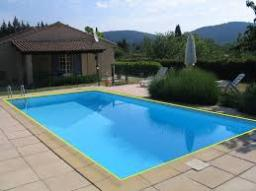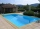# Pool tiles

The pool is 25m long, 10m wide, and 160cm deep. How many m2 of tiles will be needed on the walls and the pool? How many tiles are needed when 1 tile has a square shape with a 20cm side? How much does it cost when 1m2 of tiles costs 258 Kc?

x =  9050
y =  93396 Kc

### Step-by-step explanation:Did you find an error or inaccuracy? Feel free to write us. Thank you!#### You need to know the following knowledge to solve this word math problem:

We encourage you to watch this tutorial video on this math problem:

## Related math problems and questions:

• Children's poolChildren's pool at the swimming pool is 10m long, 5m wide and 50cm deep. Calculate: (a) how many m2 of tiles are needed for lining the perimeter walls of the pool? (b) how many hectoliters of water will fit into the pool?
• Four prismsQuestion No. 1: The prism has the dimensions a = 2.5 cm, b = 100 mm, c = 12 cm. What is its volume? a) 3000 cm2 b) 300 cm2 c) 3000 cm3 d) 300 cm3 Question No.2: The prism base is a rhombus with a side length of 30 cm and a height of 27 cm. The height of t
• PaintingThe room is long 50 meters and wide 60dm and 300 cm high. Calculate how much it will cost painting if area of windows and doors is 15% of the total area. One square meter cost 50cents.
• TableclothsThe restaurant has sixty-two square tablecloths with a side length of 150 cm and 36 rectangular tablecloths with dimensions of 140 cm and 160 cm. A) How many meters of hemming ribbon will be needed if we add 50 cm to each tablecloth? B) The ribbon sale in
• PoolThe swimming pool is 10 m wide and 8 m long, and 153 cm deep. How many hectoliters of water are in it if the water is 30 cm below its upper edge?
• PoolThe prism-shaped pool is 2 m deep with a bottom of the isosceles trapezoid with base dimensions of 10 m and 18 m and arm legs 7 m long and 5.7 m long. During the spring cleaning, the bottom and walls of the pool must be painted. How many m2 of paint shoul
• SeedsThe field has a rectangular shape with dimensions of 128 m and 350 m. How many kg of seed are needed for sowing if the 1 m2 will consume 25 g of seeds?
• Insulate houseThe property owner wants to insulate his house. The house has these dimensions 12, and 12 m is 15 m high. The windows have 6 with dimensions 170 and 150 cm. Entrance doors are 250 and 170 cm in size. How many square meters of polystyrene does he need?
• Swimming poolA swimming pool 30 meters long is filled with water to a depth of 1 meter at the shallow end, and 5 meters at the deep end and abcd the vertical area of the pool has the shape of a trapezium with the area given by S(abcd)= 1/2 (ab + cd) x ad. What is the
• SquareCalculate the area of the square shape of the isosceles triangle with the arms 50m and the base 60m. How many tiles are used to pave the square if the area of one tile is 25 dm2?
• The cuboidThe cuboid is full to the brim with water. The external dimensions are 95 cm, 120 cm, and 60 cm. The thickness of all walls and the bottom is 5 cm. How many liters of water fit into the cuboid?
• BasenHow many square meters of tiles we need to tile the walls and floor of the pool 15 meters long, six meters wide and two meters?deep
• The roomThe room has a cuboid shape with dimensions: length 50m and width 60dm and height 300cm. Calculate how much this room will cost paint (a floor is not painted) if the window and door area is 15% of the total area and 1m2 cost 15 euro.
• Water levelWhat is the area of the water level of the pool, if after filling 25 m3 of water level by 10 cm? a) 25 m2 b) 250 m2 c) 2500 dm2 d) 25,000 cm2
• Cloth / textileWe have cloth measure 16 square meters. How many 20 cm by 20 cm by 8 cm bags you can make? Assume bag is a cuboid without one top base.
• How muchHow much money will we pay for 20 planks 4m long, 15cm wide and 26mm thick when 1m³ of wood costs 4500kč?
• Two sides paintThe door has the shape of a rectangle with dimensions of 260cm and 170cm. How many cans of paint will be needed to paint this door if one can of paint cover 2m2 of the area? We paint the doors on both sides.